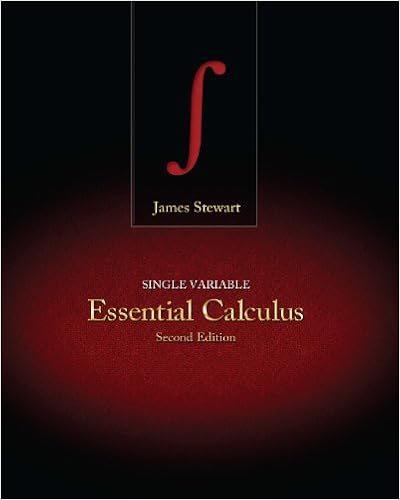# Note 2 the converse of theorem 6 is not true in

• Notes
• mimi304
• 96

This preview shows page 21 - 24 out of 96 pages.

##### We have textbook solutions for you!
The document you are viewing contains questions related to this textbook.The document you are viewing contains questions related to this textbook.
Chapter 8 / Exercise 8
Single Variable Essential Calculus
StewartExpert Verified
| NOTE 2 The converse of Theorem 6 is not true in general. If , we can- not conclude that is convergent. Observe that for the harmonic series we have as , but we showed in Example 8 that is divergent. Test for Divergence If does not exist or if , then the series is divergent. The Test for Divergence follows from Theorem 6 because, if the series is not divergent, then it is convergent, and so . Show that the series diverges. SOLUTION So the series diverges by the Test for Divergence. NOTE 3 If we find that , we know that is divergent. If we find that , we know nothing about the convergence or divergence of . Remember the warning in Note 2: If , the series might converge or it might diverge. Theorem If and are convergent series, then so are the series (where is a constant), , and , and (i) (ii) (iii) These properties of convergent series follow from the corresponding Limit Laws for Sequences in Section 11.1. For instance, here is how part (ii) of Theorem 8 is proved: Let s n lim n l s n s n 1 l n l lim n l s n 1 s lim n l a n lim n l s n s n 1 lim n l s n lim n l s n 1 s s 0 a n s n a n a n s n s a n lim n l a n 0 a n 1 n a n 1 n l 0 n l 1 n 7 lim n l a n lim n l a n 0 n 1 a n lim n l a n 0 n 1 n 2 5 n 2 4 lim n l a n lim n l n 2 5 n 2 4 lim n l 1 5 4 n 2 1 5 0 lim n l a n 0 a n lim n l a n 0 a n lim n l a n 0 a n 8 a n b n ca n c a n b n a n b n n 1 ca n c n 1 a n n 1 a n b n n 1 a n n 1 b n n 1 a n b n n 1 a n n 1 b n EXAMPLE 9 t n 1 b n t n n i 1 b i s n 1 a n s n n i 1 a i a n a n s n s n 1 s n a 1 a 2 a n
##### We have textbook solutions for you!
The document you are viewing contains questions related to this textbook.The document you are viewing contains questions related to this textbook.
Chapter 8 / Exercise 8
Single Variable Essential Calculus
StewartExpert Verified
734 CHAPTER 11 The n th partial sum for the series is and, using Equation 4.2.10, we have Therefore is convergent and its sum is Find the sum of the series . SOLUTION The series is a geometric series with and , so In Example 7 we found that So, by Theorem 8, the given series is convergent and NOTE 4 A finite number of terms doesn’t affect the convergence or divergence of a series. For instance, suppose that we were able to show that the series is convergent. Since it follows that the entire series is convergent. Similarly, if it is known that the series converges, then the full series is also convergent. u n n i 1 a i b i lim n l u n lim n l n i 1 a i b i lim n l n i 1 a i n i 1 b i lim n l n i 1 a i lim n l n i 1 b i lim n l s n lim n l t n s t a n b n n 1 a n b n s t n 1 a n n 1 b n a n b n n 1 3 n n 1 1 2 n 1 2 n a 1 2 r 1 2 n 1 1 2 n 1 2 1 1 2 1 n 1 1 n n 1 1 n 1 3 n n 1 1 2 n 3 n 1 1 n n 1 n 1 1 2 n 3 1 1 4 n 4 n n 3 1 n 1 n n 3 1 1 2 2 9 3 28 n 4 n n 3 1 n 1 n n 3 1 n N 1 a n n 1 a n N n 1 a n n N 1 a n EXAMPLE 10
SERIES 735 1. (a) What is the difference between a sequence and a series? (b) What is a convergent series? What is a divergent series? 2. Explain what it means to say that . 3–4 Calculate the sum of the series whose partial sums are given. 3. 4. 5–8 Calculate the first eight terms of the sequence of partial sums correct to four decimal places. Does it appear that the series is convergent or divergent?
•••